Publicité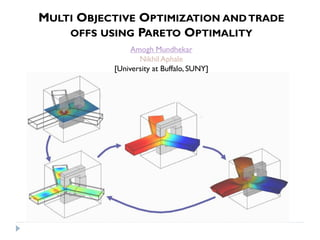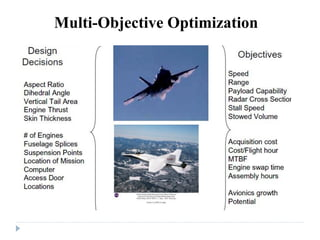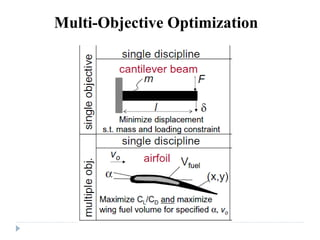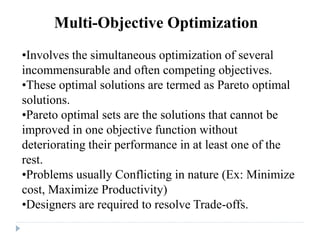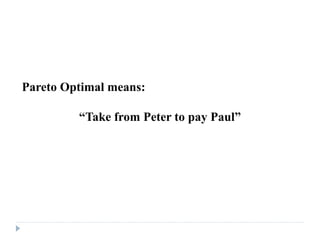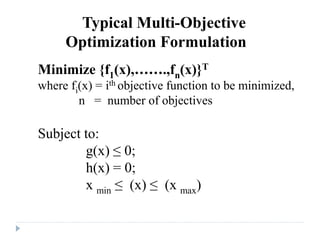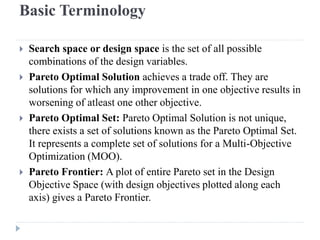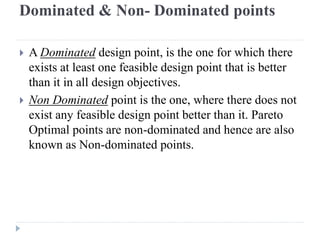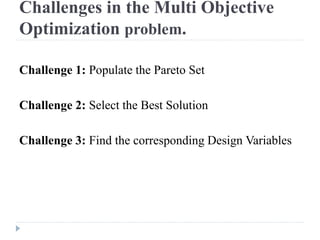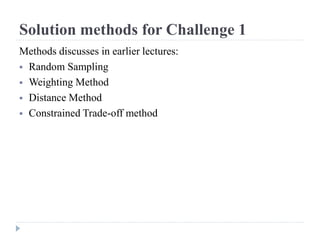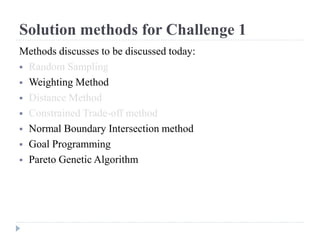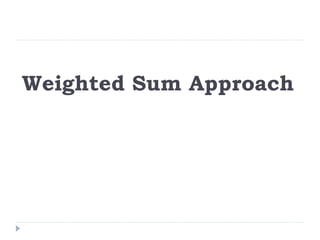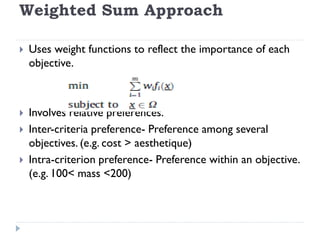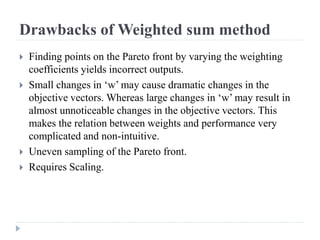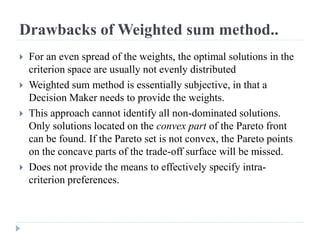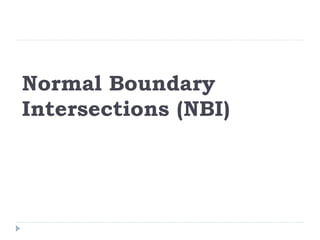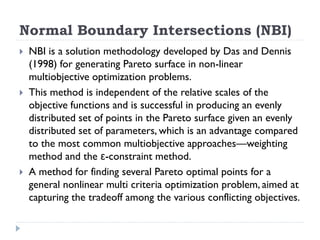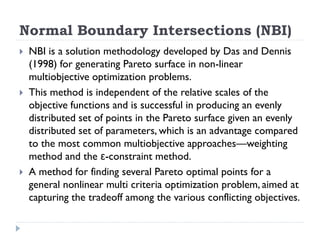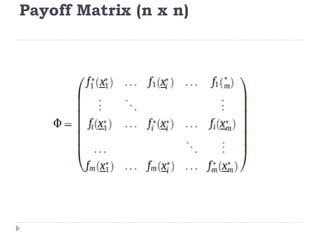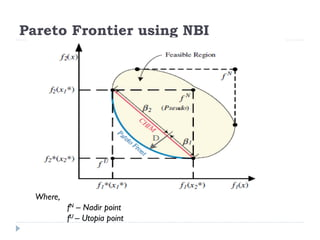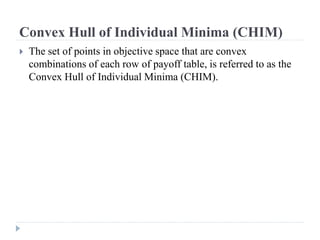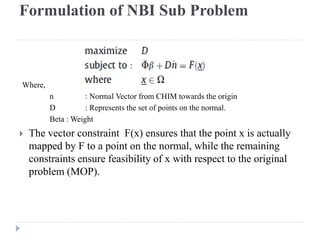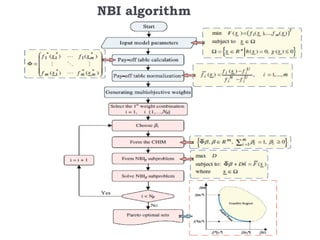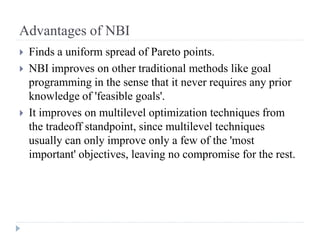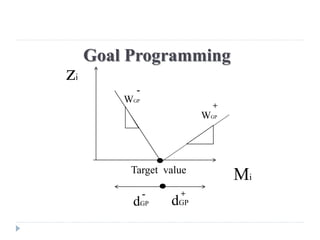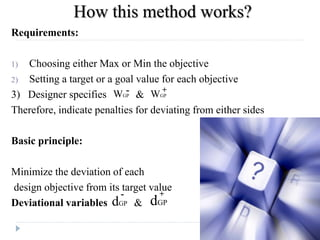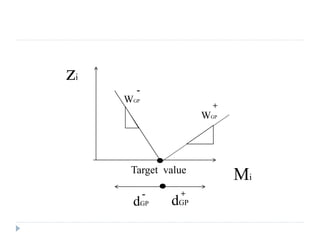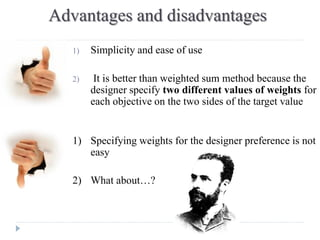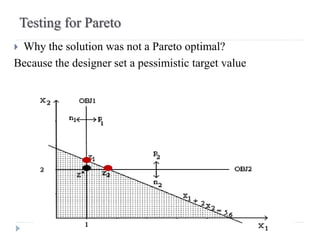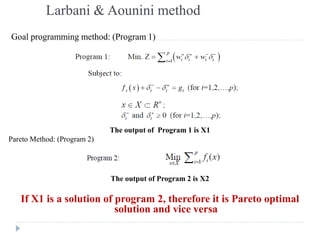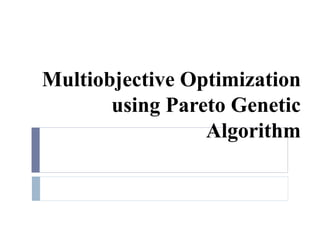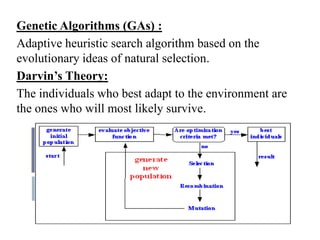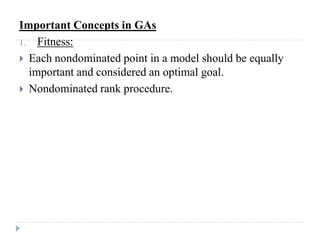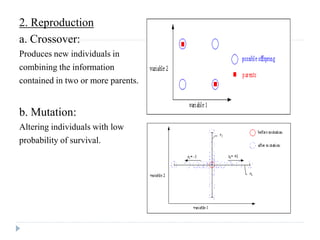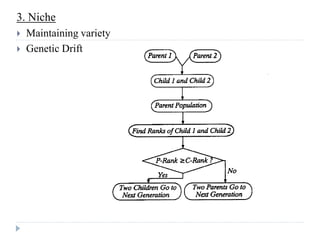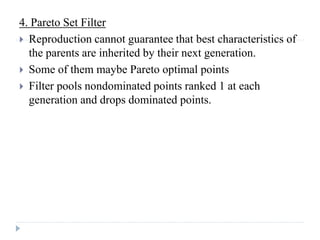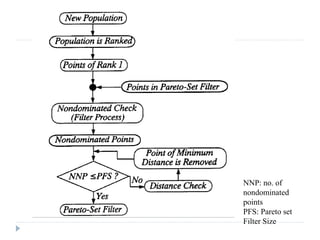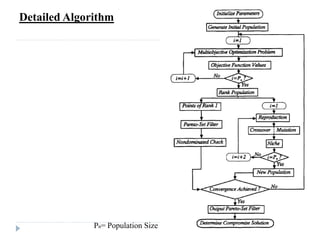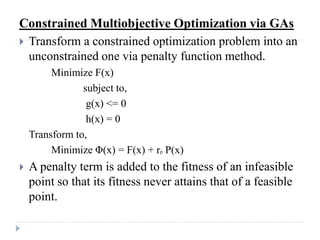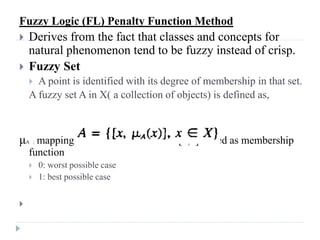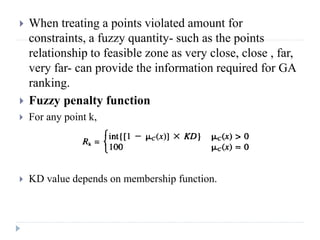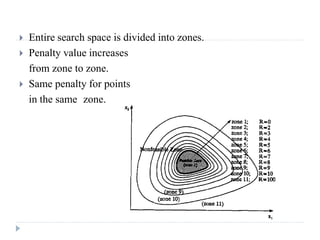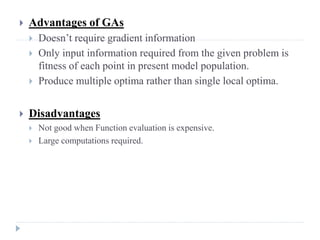1 sur 45
Publicité

### Multiobjective optimization and trade offs using pareto optimality

1. MULTI OBJECTIVE OPTIMIZATION AND TRADE OFFS USING PARETO OPTIMALITY Amogh Mundhekar Nikhil Aphale [University at Buffalo, SUNY]
2. Multi-Objective Optimization
3. Multi-Objective Optimization
4. Multi-Objective Optimization •Involves the simultaneous optimization of several incommensurable and often competing objectives. •These optimal solutions are termed as Pareto optimal solutions. •Pareto optimal sets are the solutions that cannot be improved in one objective function without deteriorating their performance in at least one of the rest. •Problems usually Conflicting in nature (Ex: Minimize cost, Maximize Productivity) •Designers are required to resolve Trade-offs.
5. Pareto Optimal means: “Take from Peter to pay Paul”
6. Typical Multi-Objective Optimization Formulation Minimize {f1(x),…….,fn(x)}T where fi(x) = ith objective function to be minimized, n = number of objectives Subject to: g(x) ≤ 0; h(x) = 0; x min ≤ (x) ≤ (x max)
7. Basic Terminology  Search space or design space is the set of all possible combinations of the design variables.  Pareto Optimal Solution achieves a trade off. They are solutions for which any improvement in one objective results in worsening of atleast one other objective.  Pareto Optimal Set: Pareto Optimal Solution is not unique, there exists a set of solutions known as the Pareto Optimal Set. It represents a complete set of solutions for a Multi-Objective Optimization (MOO).  Pareto Frontier: A plot of entire Pareto set in the Design Objective Space (with design objectives plotted along each axis) gives a Pareto Frontier.
8. Dominated & Non- Dominated points  A Dominated design point, is the one for which there exists at least one feasible design point that is better than it in all design objectives.  Non Dominated point is the one, where there does not exist any feasible design point better than it. Pareto Optimal points are non-dominated and hence are also known as Non-dominated points.
9. Challenges in the Multi Objective Optimization problem. Challenge 1: Populate the Pareto Set Challenge 2: Select the Best Solution Challenge 3: Find the corresponding Design Variables
10. Solution methods for Challenge 1 Methods discusses in earlier lectures:  Random Sampling  Weighting Method  Distance Method  Constrained Trade-off method
11. Solution methods for Challenge 1 Methods discusses to be discussed today:  Random Sampling  Weighting Method  Distance Method  Constrained Trade-off method  Normal Boundary Intersection method  Goal Programming  Pareto Genetic Algorithm
12. Weighted Sum Approach
13. Weighted Sum Approach  Uses weight functions to reflect the importance of each objective.  Involves relative preferences.  Inter-criteria preference- Preference among several objectives. (e.g. cost > aesthetique)  Intra-criterion preference- Preference within an objective. (e.g. 100< mass <200)
14. Drawbacks of Weighted sum method  Finding points on the Pareto front by varying the weighting coefficients yields incorrect outputs.  Small changes in ‘w’ may cause dramatic changes in the objective vectors. Whereas large changes in ‘w’ may result in almost unnoticeable changes in the objective vectors. This makes the relation between weights and performance very complicated and non-intuitive.  Uneven sampling of the Pareto front.  Requires Scaling.
15. Drawbacks of Weighted sum method..  For an even spread of the weights, the optimal solutions in the criterion space are usually not evenly distributed  Weighted sum method is essentially subjective, in that a Decision Maker needs to provide the weights.  This approach cannot identify all non-dominated solutions. Only solutions located on the convex part of the Pareto front can be found. If the Pareto set is not convex, the Pareto points on the concave parts of the trade-off surface will be missed.  Does not provide the means to effectively specify intra- criterion preferences.
16. Normal Boundary Intersections (NBI)
17. Normal Boundary Intersections (NBI)  NBI is a solution methodology developed by Das and Dennis (1998) for generating Pareto surface in non-linear multiobjective optimization problems.  This method is independent of the relative scales of the objective functions and is successful in producing an evenly distributed set of points in the Pareto surface given an evenly distributed set of parameters, which is an advantage compared to the most common multiobjective approaches—weighting method and the ε-constraint method.  A method for finding several Pareto optimal points for a general nonlinear multi criteria optimization problem, aimed at capturing the tradeoff among the various conflicting objectives.
18. Normal Boundary Intersections (NBI)  NBI is a solution methodology developed by Das and Dennis (1998) for generating Pareto surface in non-linear multiobjective optimization problems.  This method is independent of the relative scales of the objective functions and is successful in producing an evenly distributed set of points in the Pareto surface given an evenly distributed set of parameters, which is an advantage compared to the most common multiobjective approaches—weighting method and the ε-constraint method.  A method for finding several Pareto optimal points for a general nonlinear multi criteria optimization problem, aimed at capturing the tradeoff among the various conflicting objectives.
19. Payoff Matrix (n x n)
20. Pareto Frontier using NBI Where, fN – Nadir point fU – Utopia point
21. Convex Hull of Individual Minima (CHIM)  The set of points in objective space that are convex combinations of each row of payoff table, is referred to as the Convex Hull of Individual Minima (CHIM).
22. Formulation of NBI Sub Problem Where, n : Normal Vector from CHIM towards the origin D : Represents the set of points on the normal. Beta : Weight  The vector constraint F(x) ensures that the point x is actually mapped by F to a point on the normal, while the remaining constraints ensure feasibility of x with respect to the original problem (MOP).
23. NBI algorithm
24. Advantages of NBI  Finds a uniform spread of Pareto points.  NBI improves on other traditional methods like goal programming in the sense that it never requires any prior knowledge of 'feasible goals'.  It improves on multilevel optimization techniques from the tradeoff standpoint, since multilevel techniques usually can only improve only a few of the 'most important' objectives, leaving no compromise for the rest.
25. Goal Programming zi - WGP + WGP Target value Mi - + dGP dGP
26. History Goal programming was first used by Charnes and Cooper in 1955. The first engineering application of goal programming, was by Ignizio in 1962: Designing and placing of the antennas on the second stage of the Saturn V.
27. How this method works? Requirements: 1) Choosing either Max or Min the objective 2) Setting a target or a goal value for each objective 3) Designer specifies - WGP & WGP + Therefore, indicate penalties for deviating from either sides Basic principle: Minimize the deviation of each design objective from its target value - + Deviational variables dGP & dGP
28. zi - WGP + WGP Target value Mi - + dGP dGP
29. The Game
30. Advantages and disadvantages 1) Simplicity and ease of use 2) It is better than weighted sum method because the designer specify two different values of weights for each objective on the two sides of the target value 1) Specifying weights for the designer preference is not easy 2) What about…?
31. Testing for Pareto Why the solution was not a Pareto optimal? Because the designer set a pessimistic target value
32. Larbani & Aounini method Goal programming method: (Program 1) The output of Program 1 is X1 Pareto Method: (Program 2) The output of Program 2 is X2 If X1 is a solution of program 2, therefore it is Pareto optimal solution and vice versa
33. Multiobjective Optimization using Pareto Genetic Algorithm
34. Genetic Algorithms (GAs) : Adaptive heuristic search algorithm based on the evolutionary ideas of natural selection. Darvin’s Theory: The individuals who best adapt to the environment are the ones who will most likely survive.
35. Important Concepts in GAs 1. Fitness:  Each nondominated point in a model should be equally important and considered an optimal goal.  Nondominated rank procedure.
36. 2. Reproduction a. Crossover: Produces new individuals in combining the information contained in two or more parents. b. Mutation: Altering individuals with low probability of survival.
37. 3. Niche  Maintaining variety  Genetic Drift
38. 4. Pareto Set Filter  Reproduction cannot guarantee that best characteristics of the parents are inherited by their next generation.  Some of them maybe Pareto optimal points  Filter pools nondominated points ranked 1 at each generation and drops dominated points.
39. NNP: no. of nondominated points PFS: Pareto set Filter Size
40. Detailed Algorithm Pn= Population Size
41. Constrained Multiobjective Optimization via GAs  Transform a constrained optimization problem into an unconstrained one via penalty function method. Minimize F(x) subject to, g(x) <= 0 h(x) = 0 Transform to, Minimize Φ(x) = F(x) + rp P(x)  A penalty term is added to the fitness of an infeasible point so that its fitness never attains that of a feasible point.
42. Fuzzy Logic (FL) Penalty Function Method  Derives from the fact that classes and concepts for natural phenomenon tend to be fuzzy instead of crisp.  Fuzzy Set  A point is identified with its degree of membership in that set. A fuzzy set A in X( a collection of objects) is defined as, μ mapping from X to unit interval [0,1] called as membership A : function  0: worst possible case  1: best possible case 
43. When treating a points violated amount for constraints, a fuzzy quantity- such as the points relationship to feasible zone as very close, close , far, very far- can provide the information required for GA ranking.  Fuzzy penalty function  For any point k,  KD value depends on membership function.
44. Entire search space is divided into zones.  Penalty value increases from zone to zone.  Same penalty for points in the same zone.
45. Advantages of GAs  Doesn’t require gradient information  Only input information required from the given problem is fitness of each point in present model population.  Produce multiple optima rather than single local optima.  Disadvantages  Not good when Function evaluation is expensive.  Large computations required.
Publicité